# AP Calculus BC Practice Test 10

### Test Information8 questions24 minutes

Calculator Allowed

1.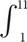f(x) dx = 24 and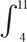f(x) dx = 20 find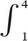f(x) dx

2. Using the Taylor series about x = 0 for sin x, approximate sin(0.4) to four decimal places.

3. Let R be the region in the first quadrant between the graphs of y = e–x, y = sin x, and the y-axis. The volume of the solid that results when R is revolved about the x-axis is

4. Use Euler’s Method, with h = 0.2 to estimate y(3), if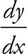= 2y - 4x and y(2) = 6.

5.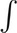sec4 x dx =

6. Let f(x) =cot x dx; 0 < x < π. If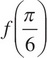= 1, then f(1) =

7.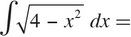8. A force of 250 N is required to stretch a spring 5 m from rest. Using Hooke’s law, F = kx, how much work, in Joules, is required to stretch the spring 7 m from rest?